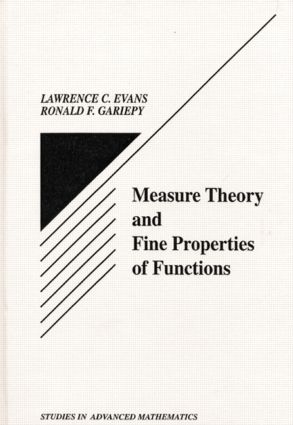# Measure Theory and Fine Properties of Functions

## 1st Edition

CRC Press

288 pages

##### Purchasing Options:\$ = USD
Hardback: 9780849371578
pub: 1991-12-18
SAVE ~\$27.00
\$135.00
\$108.00
x
eBook (VitalSource) : 9780203747940
pub: 2018-04-27
from \$28.98

FREE Standard Shipping!

### Description

This book provides a detailed examination of the central assertions of measure theory in n-dimensional Euclidean space and emphasizes the roles of Hausdorff measure and the capacity in characterizing the fine properties of sets and functions. Topics covered include a quick review of abstract measure theory, theorems and differentiation in Mn, lower Hausdorff measures, area and coarea formulas for Lipschitz mappings and related change-of-variable formulas, and Sobolev functions and functions of bounded variation.

The text provides complete proofs of many key results omitted from other books, including Besicovitch's Covering Theorem, Rademacher's Theorem (on the differentiability a.e. of Lipschitz functions), the Area and Coarea Formulas, the precise structure of Sobolev and BV functions, the precise structure of sets of finite perimeter, and Alexandro's Theorem (on the twice differentiability a.e. of convex functions).

Topics are carefully selected and the proofs succinct, but complete, which makes this book ideal reading for applied mathematicians and graduate students in applied mathematics.

### Table of Contents

GENERAL MEASURE THEORY

Measures and Measurable Functions

Lusin's and Egoroff's Theorems

Integrals and Limit Theorems

Product Measures, Fubini's Theorem, Lebesgue Measure

Covering Theorems

Differentiation of Radon Measures

Lebesgue Points

Approximate continuity

Riesz Representation Theorem

Weak Convergence and Compactness for Radon Measures

HAUSDORFF MEASURE

Definitions and Elementary Properties; Hausdorff Dimension

Isodiametric Inequality

Densities

Hausdorff Measure and Elementary Properties of Functions

AREA AND COAREA FORMULAS

Lipschitz Functions, Rademacher's Theorem

Linear Maps and Jacobians

The Area Formula

The Coarea Formula

SOBOLEV FUNCTIONS.

Definitions And Elementary Properties. Approximation

Traces. Extensions. Sobolev Inequalities

Compactness. Capacity

Quasicontinuity; Precise Representations of Sobolev Functions. Differentiability on Lines

BV FUNCTIONS AND SETS OF FINITE PERIMETER

Definitions and Structure Theorem

Approximation and Compactness

Traces. Extensions. Coarea Formula for BV Functions. Isoperimetric Inequalities.

The Reduced Boundary

The Measure Theoretic Boundary; Gauss-Green Theorem. Pointwise Properties of BV Functions

Essential Variation on Lines

A Criterion for Finite Perimeter. DIFFERENTIABILITY AND APPROXIMATION BY C1 FUNCTIONS.

Lp Differentiability a.e.; Approximate Differentiability

Differentiability A.E. for W1,P (P > N). Convex Functions

Second Derivatives a.e. for convex functions

Whitney's Extension Theorem

Approximation by C1 Functions

NOTATION

REFERENCES

### Subject Categories

##### BISAC Subject Codes/Headings:
SCI040000
SCIENCE / Mathematical Physics